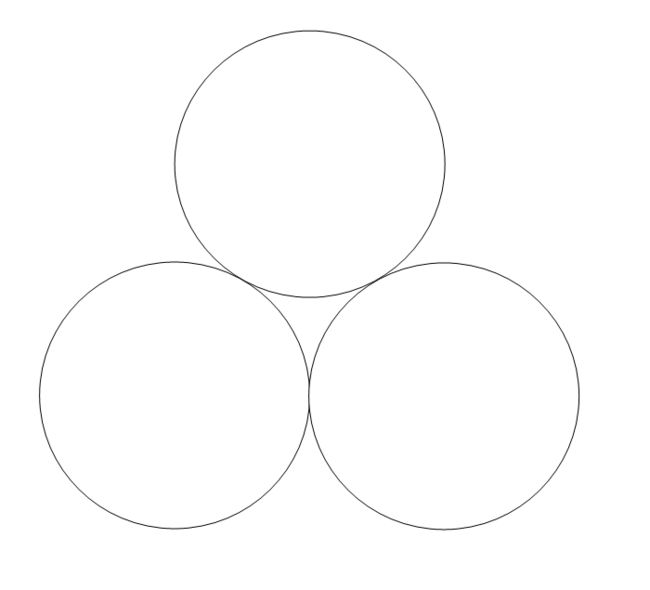# A cool-aboration Problem

Geometry Level 3Figure above shows 3 circles each with radius of 1 touching the other two circles externally.

Find the area bounded by the three circles. Give your answer to 3 decimal places.

Use the approximation $\pi=\dfrac{22}{7}$.

×